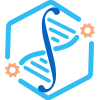### Mathematical Biosciences and Engineering

2010, Issue 2: 363-384. doi: 10.3934/mbe.2010.7.363

# Mathematically modeling PCR: An asymptotic approximation with potential for optimization

• Received: 01 April 2008 Accepted: 29 June 2018 Published: 01 April 2010
• MSC : Primary: 74G10, 92C45.

• A mathematical model for PCR (Polymerase Chain Reaction) is developed using the law of mass action and simplifying assumptions regarding the structure of the reactions. Differential equations are written from the chemical equations, preserving the detail of the complementary DNA single strand being extended one base pair at a time. The equations for the annealing stage are solved analytically. The method of multiple scales is used to approximate solutions for the extension stage, and a map is developed from the solutions to simulate PCR. The map recreates observed PCR well, and gives us the ability to optimize the PCR process. Our results suggest that dynamically optimizing the extension and annealing stages of individual samples may significantly reduce the total time for a PCR run. Moreover, we present a nearly optimal design that functions almost as well and does not depend on the specifics of a single reaction, and so would work for multi sample and multiplex applications.

Citation: Martha Garlick, James Powell, David Eyre, Thomas Robbins. Mathematically modeling PCR: An asymptotic approximation with potential for optimization[J]. Mathematical Biosciences and Engineering, 2010, 7(2): 363-384. doi: 10.3934/mbe.2010.7.363

### Related Papers:

• A mathematical model for PCR (Polymerase Chain Reaction) is developed using the law of mass action and simplifying assumptions regarding the structure of the reactions. Differential equations are written from the chemical equations, preserving the detail of the complementary DNA single strand being extended one base pair at a time. The equations for the annealing stage are solved analytically. The method of multiple scales is used to approximate solutions for the extension stage, and a map is developed from the solutions to simulate PCR. The map recreates observed PCR well, and gives us the ability to optimize the PCR process. Our results suggest that dynamically optimizing the extension and annealing stages of individual samples may significantly reduce the total time for a PCR run. Moreover, we present a nearly optimal design that functions almost as well and does not depend on the specifics of a single reaction, and so would work for multi sample and multiplex applications.###### 通讯作者: 陈斌, bchen63@163.com
• 1.

沈阳化工大学材料科学与工程学院 沈阳 1101422.080 2.1

Article outline

## Other Articles By Authors

• On This SiteDownLoad:  Full-Size Img  PowerPoint